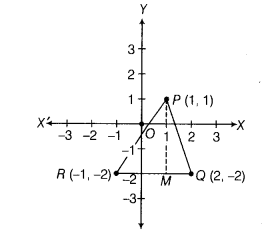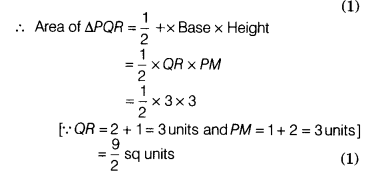# Plot the points (1,1), (2 ,-2) and (-1,-2) and check whether they are collinear or not

Plot the points (1,1), (2 ,-2) and (-1,-2) and check whether they are collinear or not. If not collinear, then determine the area of the figure.

Let us draw the coordinate axes XOX’ and YOY’ and then choose a suitable units of distance on the axes.
Let 1 cm = 1 unit.
Now, the points P(1,1), Q(2,-2)and R(-1,-2)can be plotted as shown belowOn joining these three points, we get three lines, so the given points do not lie on the same line.
So, the given points are not collinear and form a triangle. (2)
Now, draw a perpendicular line from P to RQ, i.e. PM.